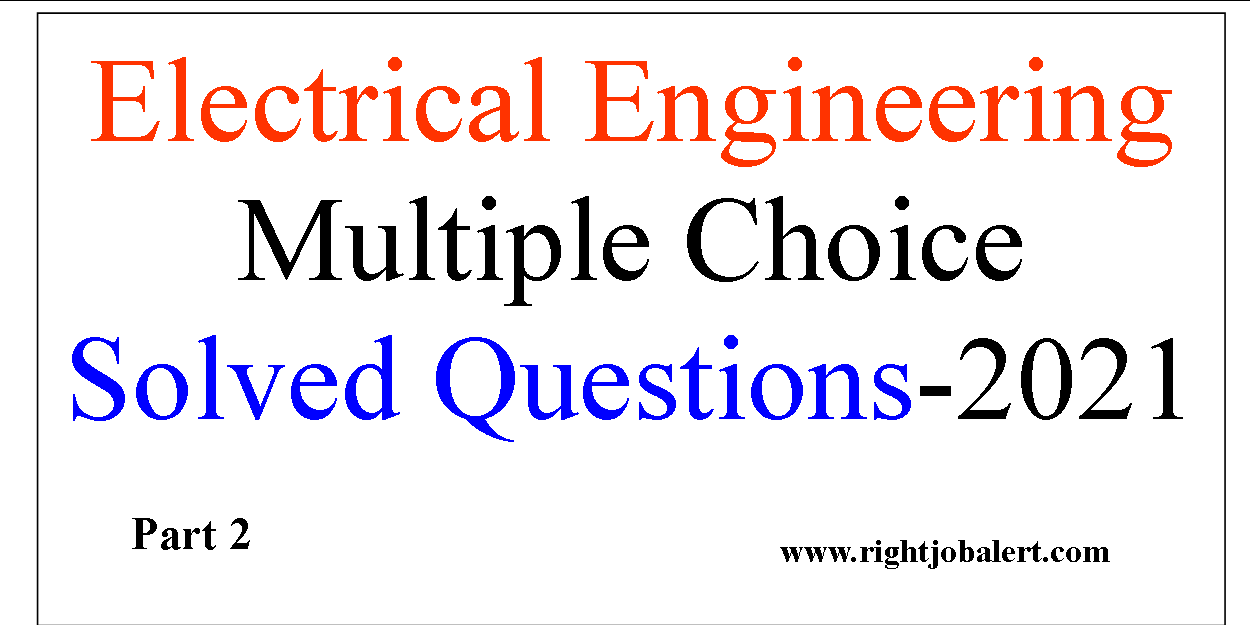## Electrical Engineering Free Multiple choice Question Answers

In Continuation of Multiple choice Electrical Engineering Solved Questions read the following Questions useful for EE Recruitment Examinations. Answers provided below each questions to store in your memory permanently. There are 15 questions below from Sl. No 11 to 25
EE Question # 11. The number of armature parallel paths in a two-pole D.C. generator having duplex lap
winding is
(a) 2
(b) 4
(c) 6
(d) 8
Ans: b

EE Question #12 . The series field of a short-shunt D.C. generator is excited by
(a) external current
(b) armature current
(c) shunt current
Ans: d

EE Question # 13. Shunt generators are most suited for stable parallel operation because of their
(a) rising voltage characteristics
(b) identical voltage characteristics
(c) drooping voltage characteristics
(d) linear voltage characteristics
Ans: c
EE Question # 14. The main factor which leads to unstable parallel operation of flat and over
compounded generators is
(a) their rising voltage characteristics
(b) unequal number of turns in their series field windings
(c) unequal speed regulation of their primemovers
(d) unequal series field resistances

EE Question # 15. Hot rolled sheets have ______ value of permeability
(a) zero
(b) low
(c) high
(d) none ofthe above

Ans: b

EE Question # 16. Two D.C. shunt generators, each with armature resistance of 0.02 ohm and field
resistance of 50 ohm run in parallel and supply a total current of 1000 amperes to the load
circuit. If their e.m.fs. are 270 V and 265 V, their bus bar voltage will be
(a) 270 V
(b) 267.5 V
(c) 265 V
(d) 257.4 V
Ans: b
EE Question # 17. The heated parts of an electrical machine dissipate heat into their surroundings by
which of the following modes of heat dissipation ?
(a) Conduction
(b) Convection
(d) All ofthe above
Ans: d

EE Question # 18. As a result of armature reaction, the reduction in the total mutual air gap flux in a D.C.
generator is approximately
(a) 40 percent
(b) 25 percent
(c) 10 percent
(d) 5 percent
Ans: d

EE Question # 19. On which of the following variables heat convected depends ?
(a) Power density
(b) Temperature difference between heated surface and coolant
(c) Thermal resistivity, density, specific heat
(d) Gravitational constant
(e) All ofthe above
Ans: eEE Question # 20. A shunt generator can self-excite
(a) only if the resistance of the field circuit is less than critical value
(b) only if the resistance of the field circuit is greater than critical value
(c) irrespective of the value of the resis-tance in the field circuit
Ans: a
EE Question # 21. Which ofthe following methods is used for air cooling of turbo-alternators ?
(a) One sided axial ventilation
(b) Two sided axial ventilation
(c) Multiple inlet system
(d) All ofthe above
Ans: d

### Basic Electrical test questions and answers PDF

EE Question # 22. For both lap and wave windings, there are as many commutator bars as the number of
(a) slots
(b) armature conductors
(c) winding elements
(d) poles
Ans: c

EE Question # 23. Multiple inlet system of air cooling of turbo-alternators can be used for machines of
rating upto
(a) 10 MW
(b) 30 MW
(c) 60 MW
(d) 150 MW
Ans: c

EE Question # 24. Which generator would you prefer for feeding long D.C. transmission lines ?
(a) Series generator
(b) Shunt generator
(c) Over compound generator
(d) Flat compound generator
Ans: c

EE Question # 25. are used for construction of core of electromagnets.
(a) Soft magnetic materials
(b) Hard magnetic materials
(c) Either (a) or (b)
(d) None of the above
Ans: a